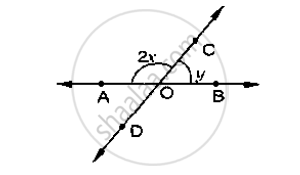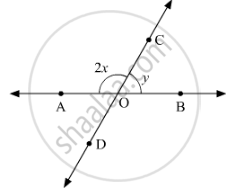# In the Below Fig, Rays Ab and Cd Intersect at O Determine X When Y =40 - Mathematics

In the below fig, rays AB and CD intersect at O

Determine x when y =40#### Solution 1

Given y = 40°, x = ?

∠AOC and ∠BOC are linear pair of angles

∠AOC + ∠BOC = 180°

⇒ 2x + y = 180°

⇒  2x + 40 = 180°

⇒ 2x = 140°

⇒ x = (140°)/2

⇒ y = 70°

#### Solution 2

Rays ABand CD intersect at point O.Therefore, ∠AOCand ∠BOCform a linear pair.

Thus,

∠AOC +∠BOC = 180°

2x +y =180°       (1)

On substituting y = 40°:

2x +y = 180°

2x + 40° = 180°

2x = 180°-40°

2x = 140°

x = (140°)/2

x = 70°

Concept: Concept of Parallel Lines
Is there an error in this question or solution?

#### APPEARS IN

RD Sharma Mathematics for Class 9
Chapter 10 Lines and Angles
Exercise 10.3 | Q 6.2 | Page 23